# Seifert conjecture

(diff) ← Older revision | Latest revision (diff) | Newer revision → (diff)

The assertion that every non-singular (i.e. everywhere non-zero)vector field on the three-dimensional spherepossesses a circular orbit.

The conjecture is a three-dimensional analogue of the well-known hairy ball theorem, stating that there is no continuous non-singular vector field on the two-dimensional sphere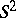.

Integrating a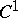vector field results in a flow, which on a closed manifoldis a dynamical system, i.e. a mapping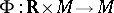with the properties:

1)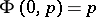; and

2)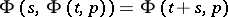(the parameteris usually interpreted as time; cf. also Dynamical system; Flow (continuous-time dynamical system)).

An orbit, or a trajectory, of a point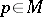is the set. If an orbit is simple closed curve, then it is called circular, closed or periodic.

The Hopf fibration is an essential mapping fromontowhose fibres, the inverse images of single points, are simple closed curves. The Seifert conjecture has its roots in a 1950 paper of H. Seifert [a8], who proved that anon-singular vector field on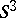possesses a periodic orbit if it is "almost parallel" to the fibres of the Hopf fibration.

The even-dimensional spheres do not admit non-singular vector fields, and a higher-dimensional version of the Seifert conjecture for the odd-dimensional spheres has been established in 1966 by F.W. Wilson [a9] as follows: Any non-singular vector field on a smooth-dimensional manifold,, can be modified to a vector field with a set of isolated invariant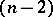-tori,, so that for:

a) in both cases as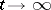and as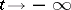, the orbit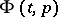limits on one of the tori; and

b) every orbit contained in one of the tori is dense in that torus. Thus, each of the spheres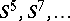admits a non-singular vector field with no circular orbits.

For his construction, Wilson introduced a plug, a special non-singular vector field on the-dimensional disc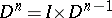, whereis the unit interval. The plug is constant and parallel to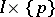on the boundary of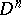, and satisfies the trapped-orbit condition and the matched-ends condition (see below). The plug can be inserted in a non-singular vector field on an-dimensional manifold (the mechanism of insertion is illustrated in Fig.a1).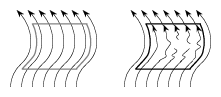Figure: s130200a

Inserting a plug

The trapped-orbit condition guarantees that at least one orbit enters the discat the bottom,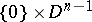, but never leaves. The matched-ends condition means that if an orbit enters the discat the bottom and leaves, then the exit point is the point onexactly above the entry point. By appropriately inserting a number of copies of a plug in a vector field on a manifold, Wilson changed the flow so that each orbit starts inside a plug and ends inside one, too.

In dimension three, Wilson's theorem yields isolated circular orbits and does not resolve the Seifert conjecture. The conjecture remained unsolved until a remarkable construction by P.A. Schweitzer in 1972. His 1974 paper [a7] describes a three-dimensional plug without periodic orbits, which Schweitzer used to break the isolated periodic orbits, see Fig.a2 and Fig.a3. Inside the plug, instead of circular orbits, there are invariant Denjoy sets to trap the entering orbits. This, initially, construction was later improved to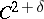by J.M. Harrison [a3].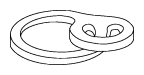Figure: s130200b

Schweitzer's plugFigure: s130200c

Breaking an orbit

Significant changes to the status of the Seifert conjecture came about in 1993 when H. Hofer [a4] proved that the Seifert conjecture holds for the Reeb vector field of a contact form on(cf. also Contact structure). It was the next, after Seifert, advancement in the spirit of the conjecture.

On the other hand, acounterexample to the Seifert conjecture (in its original formulation) was found by K. Kuperberg [a5] the same year. This aperiodic vector field onalso employs a plug. A partial self-insertion performed on a Wilson-type plug breaks the periodic orbits in the plug itself in a recursive process, see Fig.a4.Figure: s130200d

The K-plug — a self-inserted Wilson plug

The following year, G. Kuperberg [a1] modified Schweitzer's vector field to a volume-preserving counterexample to the Seifert conjecture, thereby narrowing the gap between the counterexamples and Hofer's theorem.

The above constructions generalize to higher dimensions, but counterexamples with stronger properties exist in dimensions above three. The Hamiltonian version of the Seifert conjecture is false for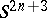for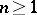, as V.L. Ginzburg [a2] proved that there is a smooth function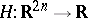,, such that the Hamiltonian flow ofonhas no closed orbits (cf. also Hamiltonian system).

## Modified Seifert conjecture.

A minimal set of a dynamical system is an invariant, non-empty, compact set containing no proper invariant, non-empty, compact subsets. The modified Seifert conjecture [a7], [a9] asserts that every non-singularvector field on an odd-dimensional sphere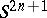,, has a minimal set of codimension at least two, i.e. of dimension at most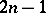. The invariant sets in the three-dimensional plugs of Wilson and Schweitzer are one-dimensional. In 1996 it was shown [a6] that the modified Seifert conjecture is false for real-analytic as well as for piecewise-linear flows, for all odd-dimensional spheres: Every non-singular vector field on any manifold can be modified in the given smoothness category so that every minimal set is of codimension one.

How to Cite This Entry:
Seifert conjecture. Encyclopedia of Mathematics. URL: http://encyclopediaofmath.org/index.php?title=Seifert_conjecture&oldid=12487
This article was adapted from an original article by K.M. Kuperberg (originator), which appeared in Encyclopedia of Mathematics - ISBN 1402006098. See original article